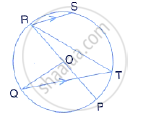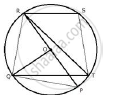Share

Books Shortlist

# The Given Figure Shows a Circle with Centre O Such that Chord Rs is Parallel to Chord Qt, Angle Prt = 20° and Angle Poq = 100°. Calculate: (I) Angle Qtr - ICSE Class 10 - Mathematics

ConceptArc and Chord Properties - If Two Arcs Subtend Equal Angles at the Center, They Are Equal, and Its Converse

#### Question

The given figure shows a circle with centre O such that chord RS is parallel to chord QT, angle PRT = 20° and angle POQ = 100°. Calculate:

(i) angle QTR#### SolutionJoin PQ, RQ and ST.

i)
∠POQ  + ∠QOR =  180°
⇒ 100° +  ∠QOR  = 180°
⇒ ∠QOR =  80°
Arc RQ subtends ∠QOR at the centre and ∠QTR at the remaining part of the circle.

∴ ∠QTR =1/2  ∠QOR

⇒ ∠QTR =  1/2 xx 80° = 40°

Is there an error in this question or solution?

#### APPEARS IN

Solution The Given Figure Shows a Circle with Centre O Such that Chord Rs is Parallel to Chord Qt, Angle Prt = 20° and Angle Poq = 100°. Calculate: (I) Angle Qtr Concept: Arc and Chord Properties - If Two Arcs Subtend Equal Angles at the Center, They Are Equal, and Its Converse.
S